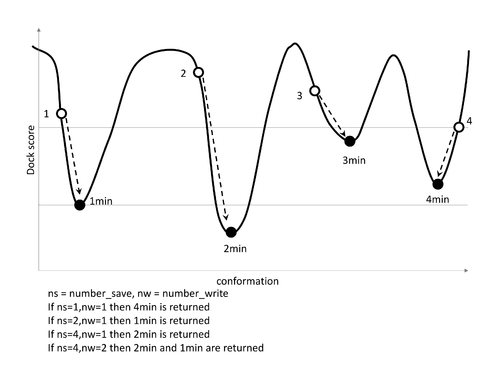# Difference between revisions of "DOCK3.7 INDOCK Minimization Parameter"

The best scored ligand conformation can be minimized using the following parameters.

Add following section to existing INDOCK after SCORING section.

```#####################################################
#                    MINIMIZATION
minimize                      yes
sim_itmax                     500
sim_trnstep                   0.2
sim_rotstep                   5.0
sim_need_to_restart           1.0
sim_cnvrge                    0.1
min_cut                       1.0e15
iseed                         777
```

The default for minimize is no. Yes turns on the minimization.

```minimize                      yes
```

How many iterations of minimization to do. More means longer run times, but potentially better poses.

```sim_itmax                     500
```

This is the initial distance in angstroms the molecule is translated (In Angstrom). (This is used to initiliaze the simplex)

```sim_trnstep                   0.2
```

How many degrees of initial rotation are done (In Degree). (This is used to initiliaze the simplex)

```sim_rotstep                   5.0
```

If the energy changes by this much, restart the minimizer from this newest position. Between 2 subseq runs: if energy changes by more than 1kcal restart minimizer from latest position for another round.

```sim_need_to_restart           1.0
```

How much the total energy can changed to be considered converged. within one run: considered converged when energies of lowest and highest energy state differ by only 0.1kcal/mol. Setting this higher will stop faster, setting it lower will cause it to do more iterations before converging (or potentially hitting the iteration max above).

```sim_cnvrge                    0.1
```

Don't minimize molecules that score above this large number.

```min_cut                       1.0e15
```

To initiliaze the simplex, this iseed number used to generate a random number. e.g. used for trn and rotstep calc

```iseed                         777
```

## Information on number_save and number_write parameters

This is true for DOCK v3.7.1rc1 (and later versions).

"number_save" is the number (for each hierarchy) that are minimized and written to OUTDOCK.

"number_write" are the number (for each hierarchy) that are written to the mol2 file.

For example, if you set number_save to 10 and number_write to 2 then 10 poses will be minimized but only the top two scoring minimized poses will be written out to the mol2 file.

The parameters (number_save/number_write) are applied to each db2 hierarchy. Each protomer/tautomer has one or more db2 hierarchies. So if there are multiple protonation state each will have the "number_write" poses written out to the mol2 file (caveat, dock might not generate that many in which case it will write all that are possible).

We recommended setting these numbers to 1 for production run discovery campaigns. we topically only modify number_save/number_write to 10 if you want to perform more exhaustive sampling for a small number (e.g. less than 1000) of molecules.how resulting poses will very with different values of number_save and number_write